My name is Tony and I'm a senior at Memorial High. My question is; When rolling 2 dice, what is the probability of rolling 5 sevens before rolling a six or an eight? Thanks, Tony Hi Tony, The probability of rolling a seven is 6/36 The probability of rolling a six is 5/36 The probability of rolling an eight is 5/36 Thus the probability of an outcome that is not a six nor an eight is 1 - 5/36 - 5/36 = 20/36 Suppose that the fifth 7 is rolled on the nth roll and none of the previous n - 1 rolls is a five or an eight. This string of n - 1 rolls then consists of 4 sevens and n - 5 outcomes that are not fives or eights. The probability of this arrangement in n - 1 rolls is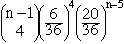The factor of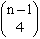is necessary since you can choose 4 of the n - 1 rolls to be sevens. n can be any positive integer larger than 4 and hence the probability of rolling 5 sevens before rolling a six or an eight is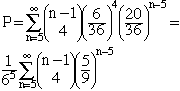If you let x = 5/9 then this expression is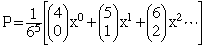You can evaluate this expression if you know Newton's form of the binomial theorem. If |x| < 1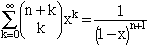Hence, for n = 4 this expression yields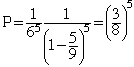Andrei and Penny Go to Math Central